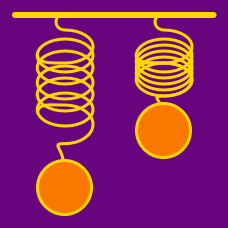Classical Mechanics

# Periodic Motion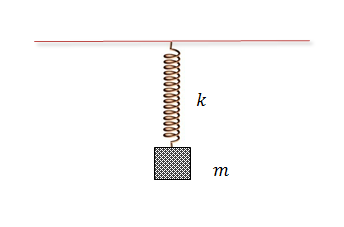A body of mass $$m = 4 \text{ kg}$$ is attached to a spring that hangs from the ceiling. If the spring is held at its original length and then let go, the body oscillates with amplitude $$A = {\frac{a}{b}} \text{ m},$$ where $$a$$ and $$b$$ are coprime positive integers. Find the value of $$a+b.$$

The spring constant is $$k = 5 \text{ N/m}$$ and the gravitational acceleration is $$g=10\text{ m/s}^2.$$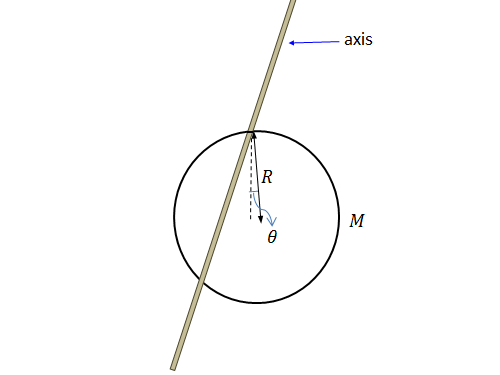A ring of mass $$M = 5 \text{ kg}$$ and radius $$R = 3 \text{ m}$$ oscillates on a horizontally placed rod. If the ring's angular frequency can be expressed as $$\omega = \sqrt{\frac{a}{b}} \text{ rad/s},$$ where $$a$$ and $$b$$ are coprime positive integers, what is the value of $$a+b?$$

The moment of inertia is given by $$I = 2MR^2$$ and the gravitational acceleration is $$g=10\text{ m/s}^2.$$A body of mass $$m = 8 \text{ kg}$$ oscillates on a spring hanging from the ceiling with an angular frequency of $$\omega = \sqrt{\frac{a}{b}} \text{ rad/s},$$ where $$a$$ and $$b$$ are coprime positive integers. Find the value of $$a+b.$$

The spring constant is $$k = 5 \text{ N/m}$$ and the gravitational acceleration is $$g=10\text{ m/s}^2.$$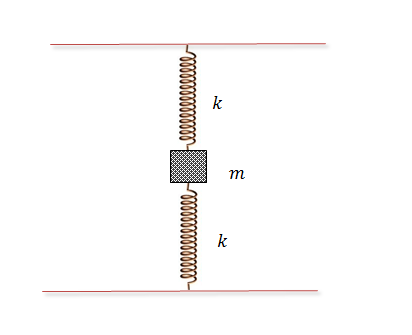In the figure above, a body of mass $$m = 4 \text{ kg}$$ oscillates in the middle of two identical springs with an angular speed of $$\omega = \sqrt{\frac{a}{b}} \text{ rad/s},$$ where $$a$$ and $$b$$ are coprime positive integers. Find the value of $$a+b.$$

The spring constant is $$k = 9 \text{ N/m}$$ and the gravitational acceleration is $$g=10\text{ m/s}^2.$$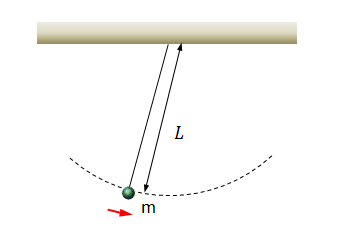A pendulum of mass $$m = 5 \text{ kg}$$ and length $$L = 12 \text{ m}$$ oscillates with an angular frequency of $$\omega = \sqrt{\frac{a}{b}} \text{ rad/s},$$ where $$a$$ and $$b$$ are coprime positive integers. Find the value of $$a+b.$$

The gravitational acceleration is $$g=10\text{ m/s}^2.$$

×

Problem Loading...

Note Loading...

Set Loading...